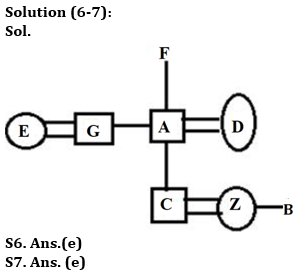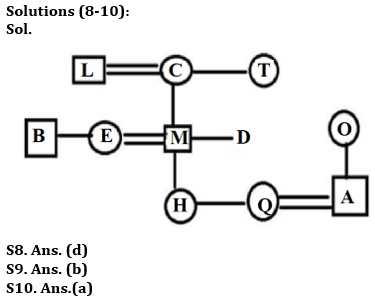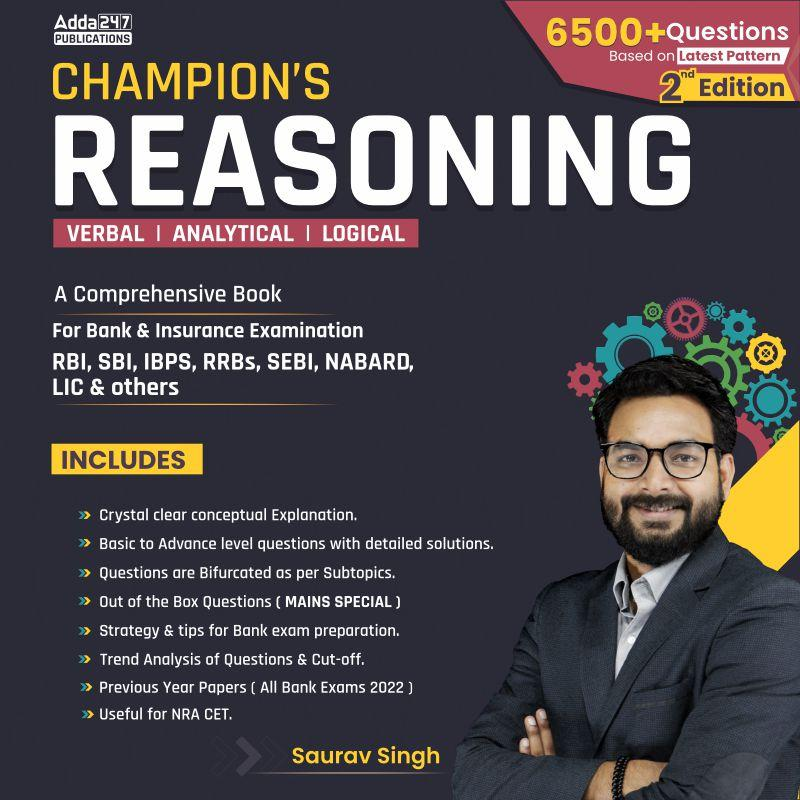Latest Banking jobs   »   IBPS reasoning

# Reasoning Quiz For IBPS Clerk Prelims 2023-28th August

Directions (1-5): In the given questions, relationship between different elements is shown in the statement(s). These statements are followed by two conclusions. Find which of the conclusion(s) will be definitely true.

Q1. Statements: L > M < N = R ≤ S, T ≥ G < W> S
Conclusions: I. R > T
II. M < W
(a) Only conclusion I is true
(b) Only conclusion II is true
(c) Either conclusion I or II is true
(d) Neither conclusion I nor II is true
(e) Both conclusions I and II are true

Q2. Statements: P ≥ Q, Q ≤ X, X > S, S < Y
Conclusions: I. P > X
II. Y > X
(a) Only conclusion I is true
(b) Only conclusion II is true
(c) Either conclusion I or II is true
(d) Neither conclusion I nor II is true
(e) Both conclusions I and II are true

Q3. Statements: N = K < E ≤ B = Q ≥ U > G
Conclusions: I. B > G
II. Q ≥ E
(a) Only conclusion I is true
(b) Only conclusion II is true
(c) Either conclusion I or II is true
(d) Neither conclusion I nor II is true
(e) Both conclusions I and II are true

Q4. Statements: L ≤ Q, N > Z, L ≤ Z, N = Y
Conclusions: I. L < Y
II. Z > Q
(a) Only conclusion I is true
(b) Only conclusion II is true
(c) Either conclusion I or II is true
(d) Neither conclusion I nor II is true
(e) Both conclusions I and II are true

Q5. Statements: W ≥ U = J, K ≤ V = S, S < M > W
Conclusions: I. M > J
II. V < W
(a) Only conclusion I is true
(b) Only conclusion II is true
(c) Either conclusion I or II is true
(d) Neither conclusion I nor II is true
(e) Both conclusions I and II are true

Directions (6-7): Study the following information carefully and answer the questions that follow.
In a three-generation family, there are eight members, Z, A, B, C, D, E, F, and G in which there are three married couples. D is the mother of C who is the brother-in-law of B. G is the paternal uncle of C. F has only two sons and both sons are married. C is the grandson of F. E is the daughter-in-law of F. D has only one child. A does not belong to the 3rd generation.

Q6. What is the relation of B with respect to Z?
(a) Wife
(b) Brother-in-law
(c) Sister-in-law
(d) Brother
(e) Can’t be determined

Q7. Who is the mother-in-law of E?
(a) Z
(b) A
(c) F
(d) D
(e) Can’t be determined

Directions (8-10): Read the following information carefully and answer the questions given below.
There are eleven members in a family of three generations in which there are three married couples. B is the brother of E who is the sister-in-law of D. M is the sibling of D Q is the female member of the family. L is the father-in-law of E. H is the granddaughter of C who is the spouse of L. H is the daughter of E. T is the sister-in-law of L. Sibling of H is the daughter-in-law of O. T is unmarried. O is the mother of A. M is one of the members of the family.

Q8. How many female members are there in the family?
(a) Six
(b) Seven
(c) Eight
(d) Either (a) or (b)
(e) None of these

Q9. What is the relation of Q with respect to O?
(a) Niece
(b) Daughter-in-law
(c) Sister-in-law
(d) Daughter
(e) None of these

Q10. Who is the grandmother of H?
(a) C
(b) T
(c) M
(d) Q
(e) O

Solutions

Solutions (1-5):
S1. Ans. (b)
Sol. I. R > T – False
II. M < W – True

S2. Ans. (d)
Sol. I. P > X – False
II. Y > X – False

S3. Ans. (e)
Sol. I. B > G – True
II. Q ≥ E – True

S4. Ans. (a)
Sol. I. L < Y – True
II. Z > Q – False

S5. Ans. (a)
Sol. I. M > J – True
II. V < W – False## FAQs

### What is the selection process of IBPS Clerk 2023

The selection process of IBPS Clerk includes Prelims and Mains exam

#### Congratulations!Union Budget 2023-24: Free PDF